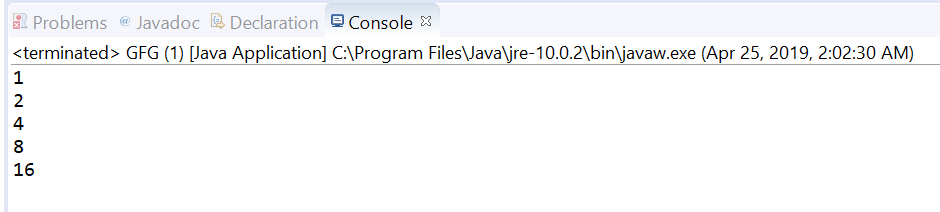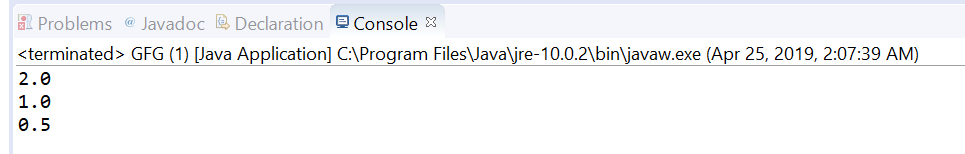# Stream iterate(T,Predicate,UnaryOperator) method in Java with examples

• Last Updated : 25 Apr, 2019

The iterate(T, java.util.function.Predicate, java.util.function.UnaryOperator) method allows us to iterate stream elements till the specified condition. This method returns a sequential ordered Stream produced by iterative application of the given next function to an initial element, conditioned on satisfying hasNext predicate passed as parameter. The stream terminates as soon as the hasNext predicate returns false.

The resulting sequence returned by this method may be empty if passed predicate does not hold on the seed value. Otherwise, the first element will be the supplied seed value, the next element will be the result of applying the next function to the seed value, and so on iteratively until the hasNext predicate indicates that the stream should terminate.

Syntax:

```static <T> Stream<T> iterate(T seed,
Predicate<T> hasNext,
UnaryOperator<T> next)
```

Parameters: This method accepts three parameters:

• seed: which is the initial element,
• hasNext: which is a predicate to apply to elements to determine when the stream must terminate and
• next: which is a function to be applied to the previous element to produce a new element.

Return value: This method returns a new sequential Stream.

Below programs illustrate iterate(T, java.util.function.Predicate, java.util.function.UnaryOperator) method:

Program 1:

 `// Java program to demonstrate``// Stream.iterate method`` ` `import` `java.util.stream.Stream;``public` `class` `GFG {`` ` `    ``public` `static` `void` `main(String[] args)``    ``{`` ` `        ``// create a stream using iterate``        ``Stream stream``            ``= Stream.iterate(``1``,``                             ``i -> i <= ``20``, i -> i * ``2``);`` ` `        ``// print Values``        ``stream.forEach(System.out::println);``    ``}``}`

The output printed on console of IDE is shown below.
Output:Program 2:

 `// Java program to demonstrate``// Stream.iterate method`` ` `import` `java.util.stream.Stream;``public` `class` `GFG {`` ` `    ``public` `static` `void` `main(String[] args)``    ``{`` ` `        ``// create a stream using iterate``        ``Stream stream``            ``= Stream.iterate(``2.0``,``                             ``decimal -> decimal > ``0.25``, decimal -> decimal / ``2``);`` ` `        ``// print Values``        ``stream.forEach(System.out::println);``    ``}``}`

The output printed on console of IDE is shown below.
Output:My Personal Notes arrow_drop_up